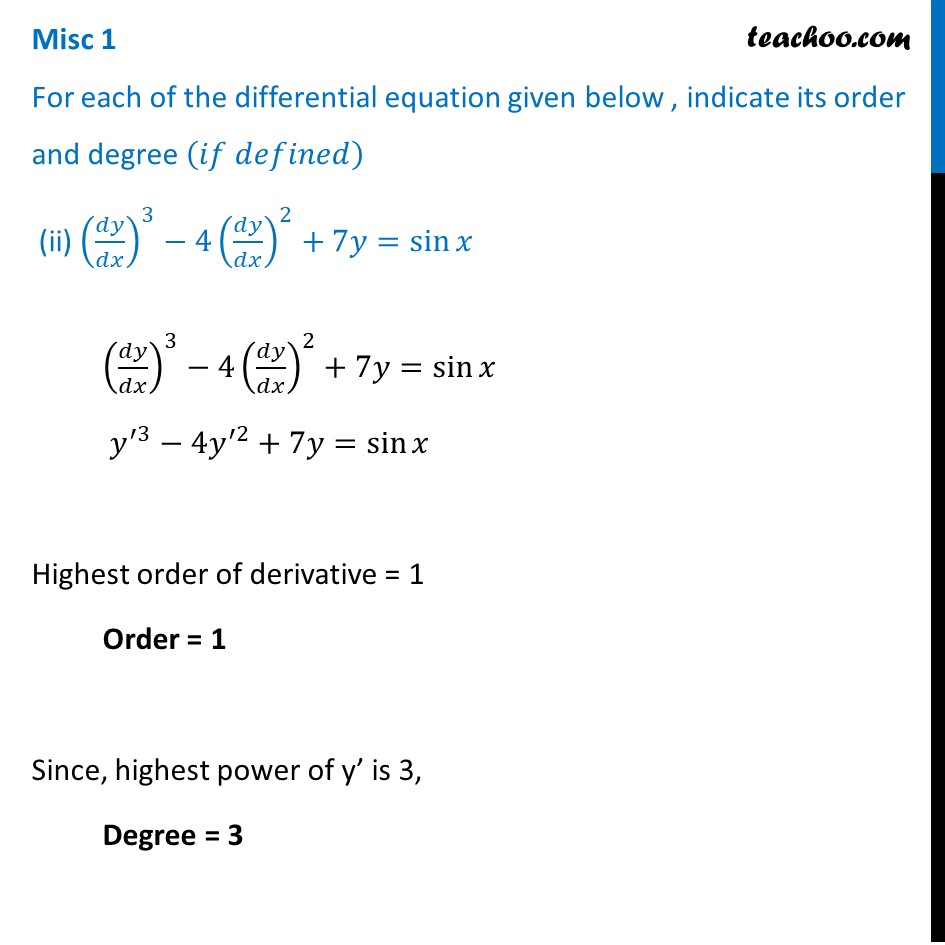Miscellaneous

Chapter 9 Class 12 Differential Equations
Serial order wiseLearn in your speed, with individual attention - Teachoo Maths 1-on-1 Class

### Transcript

Misc 1 For each of the differential equation given below , indicate its order and degree (𝑖𝑓 𝑑𝑒𝑓𝑖𝑛𝑒𝑑) (ii) (𝑑𝑦/𝑑𝑥)^3−4(𝑑𝑦/𝑑𝑥)^2+7𝑦=sin⁡𝑥 (𝑑𝑦/𝑑𝑥)^3−4(𝑑𝑦/𝑑𝑥)^2+7𝑦=sin⁡𝑥 𝑦^′3−4𝑦^′2+7𝑦=sin⁡𝑥 Highest order of derivative = 1 Order = 1 Since, highest power of y’ is 3, Degree = 3